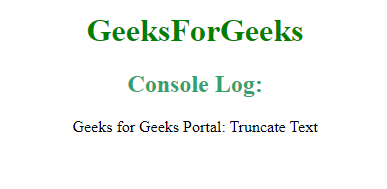Open In App

# How to truncate text in Angular2?

Approach: It is easy to truncate text in Angular. The truncated text helps us to remove or cut-off portions of text. It abruptly ends the text, reducing the length of the text. It removes text in accordance with the truncate function used.

Syntax:

• String length is greater than the given characters.
`function(str, length, ending) {........}`
• String truncates to certain words length specified.
```function truncate(str, no_words) {
return str.split(" ").splice(0, no_words).join(" ");
}```

Example 1: This example shows the way text in Angular is made truncate. Here, the length of the text/string is longer than the given characters. The truncate function is created, which checks the condition if the string length is greater than the given length. It then returns the truncated text accordingly.

 ` ``<``html``> ``<``head``> ``    ``<``title``>Truncate text in Angular2 `` ` `    ``<``script` `src` `= ``"https://ajax.googleapis.com/ajax/libs/angularjs/1.3.14/angular.min.js"``> `` ` `    ``<``script` `src` `= ``"https://ajax.googleapis.com/ajax/libs/angularjs/1.3.14/angular-route.min.js"``> ``    `` `` ``     ` `<``body` `style` `= ``"text-align:center;"``> ``         ` `    ``<``h1` `style` `= ``"color:green;"` `> ``        ``GeeksForGeeks ``    `` ``         ` `    ``<``script``> ``text_truncate = function(str, length, ending) {``      ` `    ``if (ending == null) {``      ``ending = '...';``    ``}``      ` `    ``if (str.length > length) {``      ``return str.substring(0, length - ending.length) + ending;``    ``} else {``      ``return str;``    ``}``  ``};``  ` `console.log(text_truncate('Truncate text using Geeks for Geeks',11))``console.log(text_truncate('Truncate text using Geeks for Geeks',11,'!!'))``    `` `` ``                    `

Output:Example 2: This example shows how to truncate the text to certain words. It removes the words that are not included in the given limit. For this purpose: str.split, splice and join are used.

 ` ``<``html``> ``<``head``> ``    ``<``title``>Angular JS Route Change `` ` `    ``<``script` `src` `= ``"https://ajax.googleapis.com/ajax/libs/angularjs/1.3.14/angular.min.js"``> `` ` `    ``<``script` `src` `= ``"https://ajax.googleapis.com/ajax/libs/angularjs/1.3.14/angular-route.min.js"``> ``    `` `` ``     ` `<``body` `style` `= ``"text-align:center;"``> ``         ` `    ``<``h1` `style` `= ``"color:green;"` `> ``        ``GeeksForGeeks ``    `` ``         ` `    ``<``script``> ``function truncate(str, no_words) {``    ``return str.split(" ").splice(0, no_words).join(" ");``}`` ` `console.log(truncate('Geeks for Geeks Portal: Truncate Text Using GFG', 6));``    `` `` ``                    `

Output: